变频器维修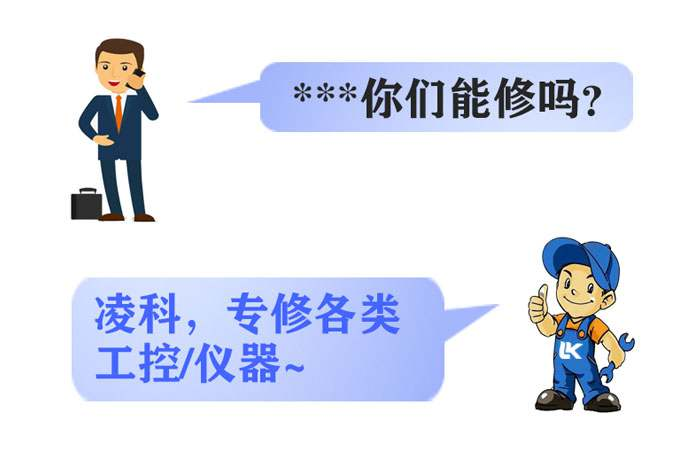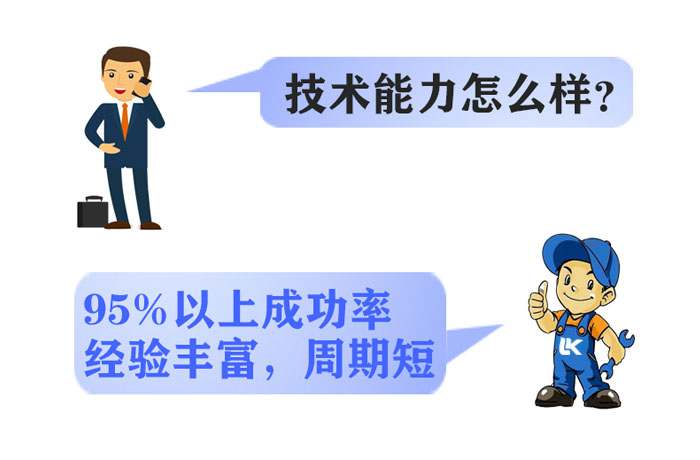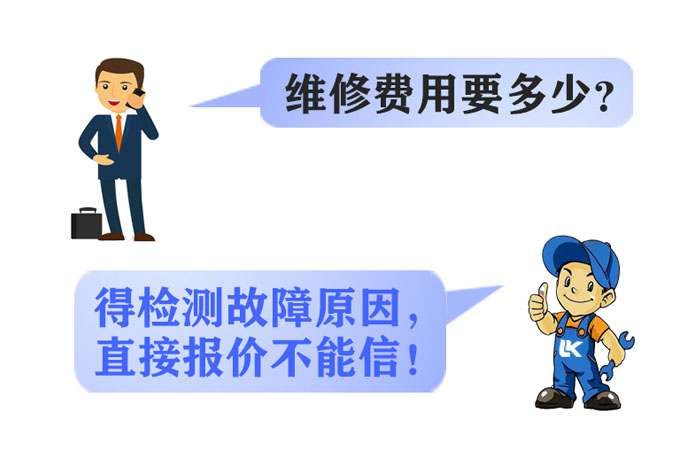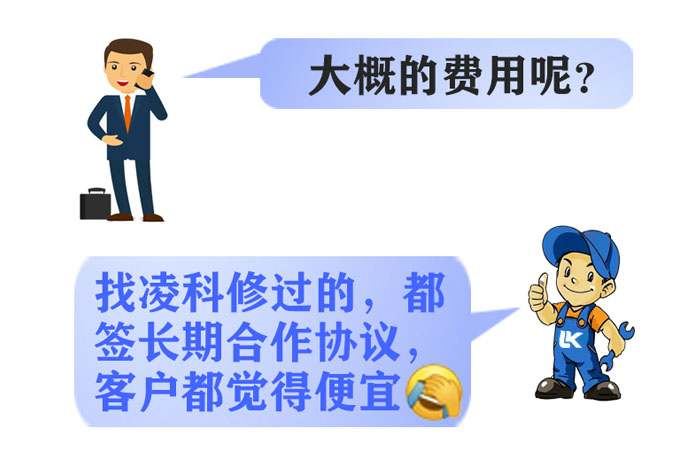西门子变频器维修中心

2020-08-26 16:44:32变频器维修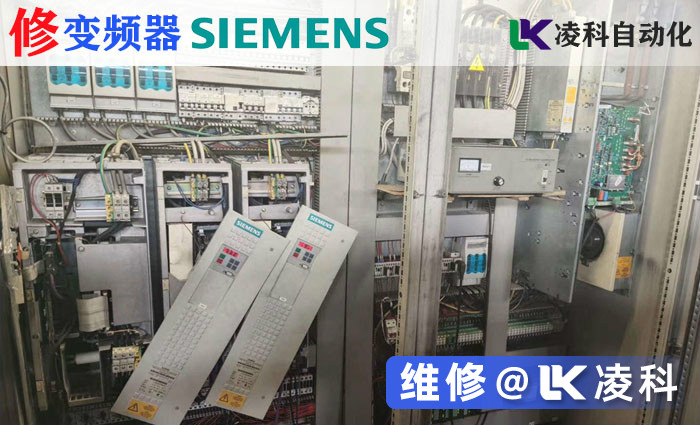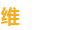13961121525（王工）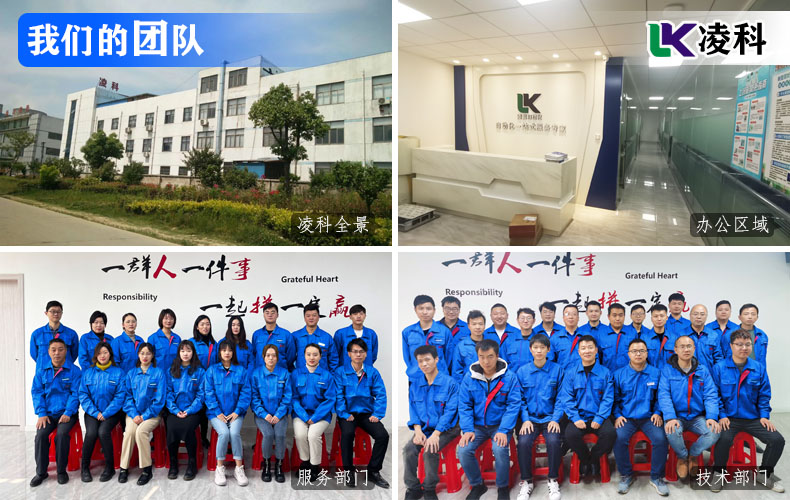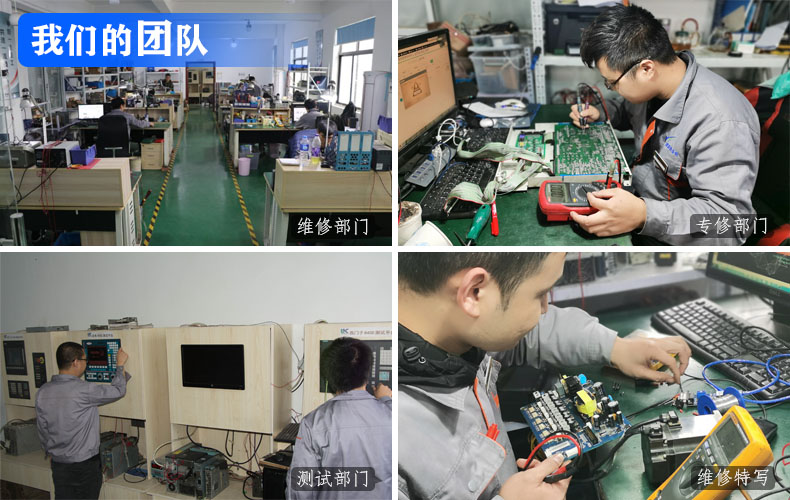13961121525（王工）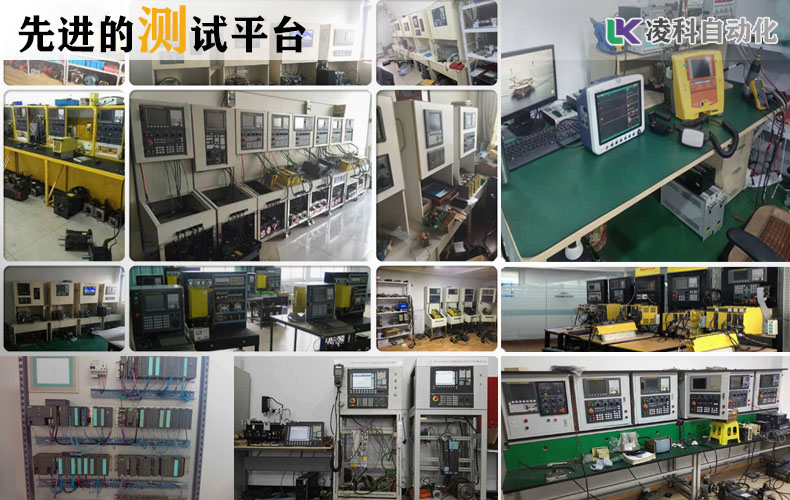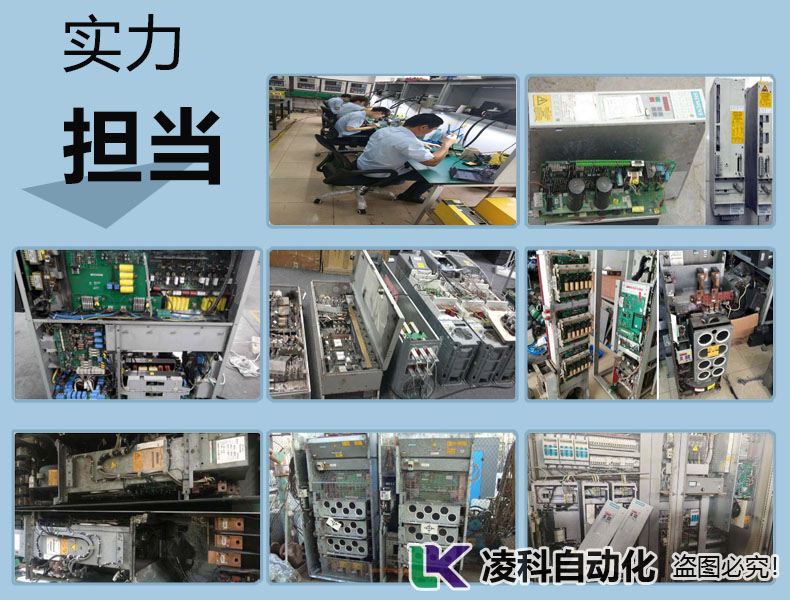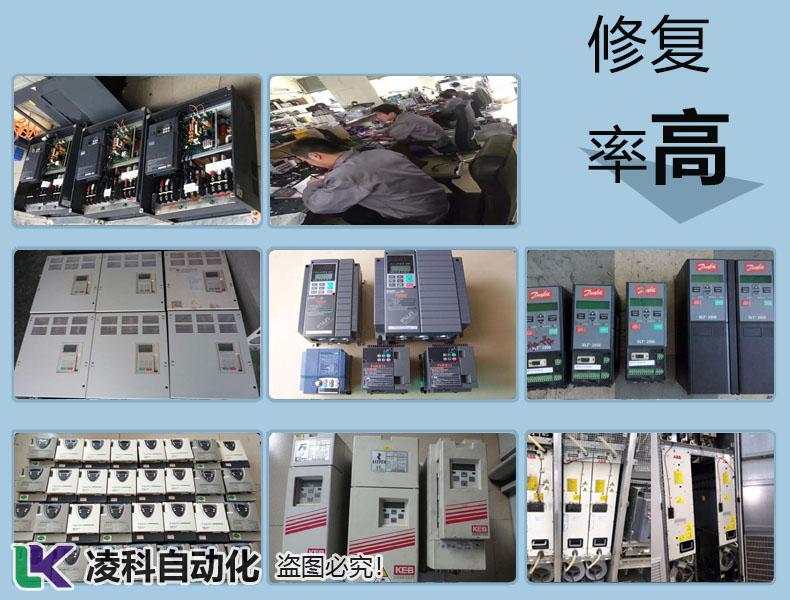13961121525（王工）

风雨同行 · 凌科与你！

20多年维修经验，60+专修大咖，300+技术服务团队！

••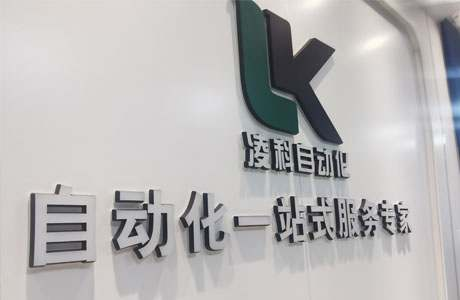总公司外景
•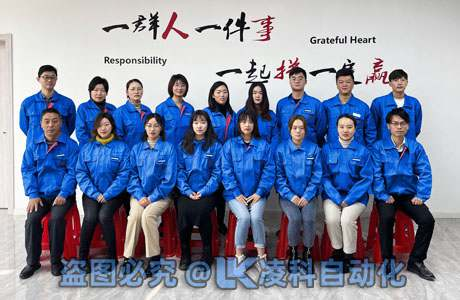我们是售后一部
•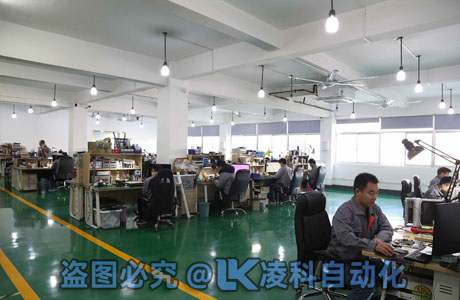维修一部现场
•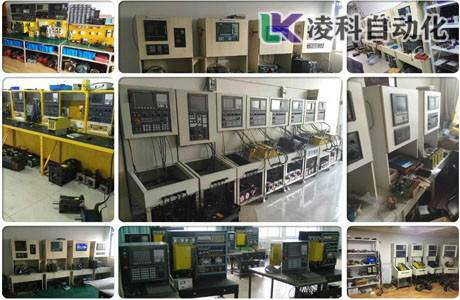维修测试平台
•工控测试平台
•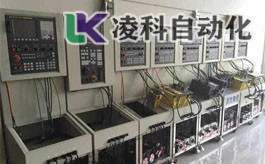测试平台展示
•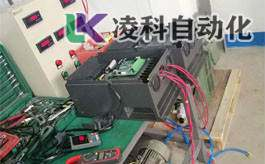负载测试平台
•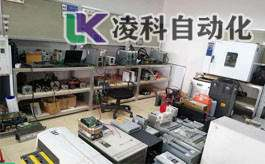工控维修二部
•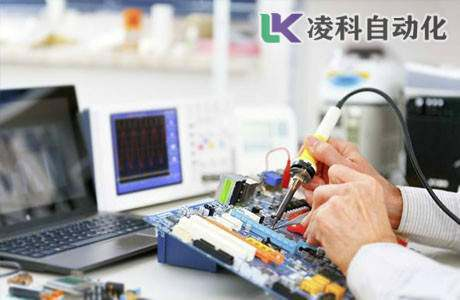维修现场特写
•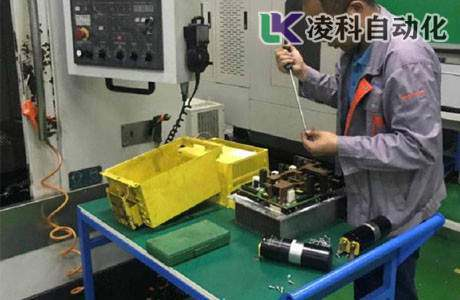现场维修特写
•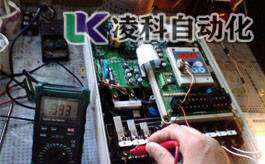维修测试现场

工控/仪器仪表维修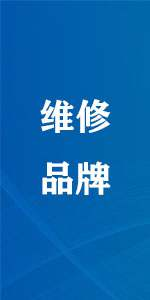• 西门子
• ABB
• 安川
• 松下
• 富士
• 施耐德
• 艾默生CT
• SEW
• 三菱
• 丹佛斯
• 台达
• 台安
• 东元
• LS(LG)
• 汇川
• 英威腾
• 海利普
• 普传
• 康沃
• 安邦信
• 易能
• 伟肯
• 东芝
• 士林
• 科比
• 伦茨Lenze
• 罗克韦尔
• 日立
• 森兰
• 伟创
• 四方
• 博世力士乐
• 正弦
• 阿尔法
• 欧姆龙
• 三垦
• 欧陆
• 派克Parker
• 发那科FANUC
• 海德汉
• 贝加莱
• 科尔摩根
• LUST
• 埃斯顿
• 海泰克
• proface
• 宝元
• 新代
• 发格FAGOR
• NUM
• 哈斯HAAS
• 大隈OKUMA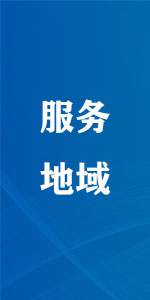华东，华南，华中，华北，东北，西北，西南，北京，上海，天津，重庆……
• 南京
• 无锡
• 徐州
• 常州
• 苏州
• 青岛
• 南通
• 连云港
• 淮安
• 盐城
• 扬州
• 镇江
• 泰州
• 宿迁
• 江阴
• 宜兴
• 张家港
• 常熟
• 丹阳
• 溧阳
• 句容
• 溧水
• 高淳
• 金坛
• 靖江

大家对凌科的认可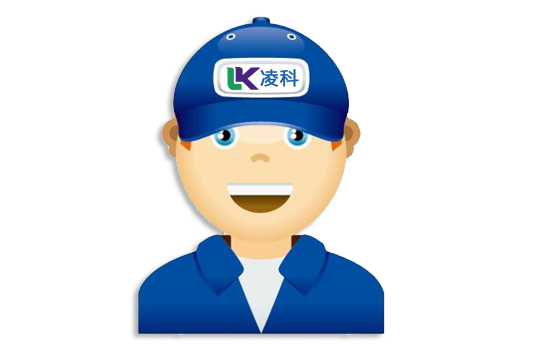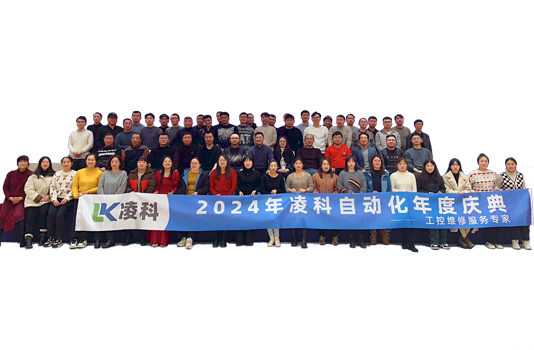x